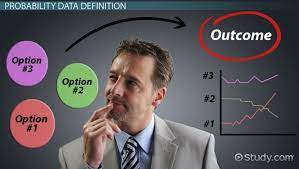## The Introduction to Probability

The future is full of many uncertainties, and the likelihood that an event would happen is between 0 and 1. The probability that success is almost certain is determined by its closeness to 1 while zero indicated that success is quite unlikely. The concept of probability theory can be applied to many situations we face every day of our lives.

The concept of the Complement of an event, the Union, the Mutually Exclusive, and the Independent of events can be applied to guide our daily decisions. A good assessment of every event, and its dependent variables could help to make the best choice in a critical situation.

Though an experiment is repeated the same way, an entirely different outcome could occur, and this is the reason it is sometimes called random experiments. There are three methods of assigning probability:

1. Classical Method. This method is used to assign probability based on the assumption of equally likely outcomes.
2. Relative Frequency Method: Probability is assigned based on experimentation or historical data
3. Subjective Method. Assigning probabilities based on judgment.

The best probability estimates are often obtained by combining the estimate from the classical or relative frequency approach with the subjective estimate.

## Complement of an Event.

The complement of an event consist of all sample points that are not in the event. In making decisions, it is imperative to consider external variables that could possibly affect the outcome of such decisions. This consideration could shift the probability of a successful outcome to a higher or lower margin.

## The Union of Two Events and Additional Law

The Union of two events (AUB) consists of all events in each of the sample points and the combination of the two. The intersection of two events (AB) is the set of all sample points that are in both events.

With additional Law, the probability of event A, or B, or Both A and B occurring can be determined by The additional law which is expressed as P(A U B) = P(A)+P(B)-P(A ∩ B)

Mutually Exclusive Events and Independent Event

Two events can be said to be mutually exclusive if, when one occurs, the other cannot occur. For example, a tossed coin outcome can either be a head or a tail, but both outcomes cannot occur simultaneously. The Mutually Exclusive event is often misunderstood as an independent event. Unlike mutually exclusive events, independent events can occur simultaneously. The independence indicates that the outcome probability of one event does not influence the outcome probability of another event. For example, you flip a coin and get ahead and you flip a second coin and get a tail. The two coins don’t influence each other.

In Mutual Exclusive event, the rule of multiplication states that since the events cannot occur simultaneously, their joint probability {P.(A B)} is always zero.

Mutually Exclusive is denoted as P( A U B)= P(A) + P(B). P(A B)=0

Event A and B can be said to be independent if P(A/B) = P(A) or P(B/A) = P(B)

## Conditional Probability and  Multiplication Law

Conditional Probability:

This is expressed as the probability of an event given that another event has previously occurred. It can be expressed as P(A|B) = P (A ∩ B)/P(B).

Multiplication Law

The multiplication law provides a way to compute the probability of the intersection of two events.

P(A ∩ B) = P(A|B) x P(B). This is expressed as the probability of A, given B has occurred multiplied by the probability of B.## Next Generation Fixed Wireless Access (NGFWA) for Service Providers: A Viable Alternative to Traditional Fiber-Optic Networks

in## Transforming Nigeria’s Local Government System for Rural Economic Growth and Increased GDP

in